Related Articles

# Tangent to a Circle

Tangent in Circles are the line segments that touch the given curve only at one particular point. Tangent is a Greek word meaning “To Touch”. For a circle, we can say that the line which touches the circle from the outside at one single point on the circumference is called the tangent of the circle A circle can have many tangents but at a particular point on the circumference of the circle, only one tangent passes through that point on the circle. The tangent to a circle is always perpendicular to the radius of the circle.

In this article, we will learn about tangent to a circle, the equation of tangent, their properties, theorems, and examples.

## What is Tangent to a Circle?

We define tangent as the line drawn from the external point that passes through a particular point on the curve. Examples of tangents are the lines that represent the velocity of the particle performing uniform circular motion at any instant. We can have many tangents to a curve but from a particular point, only a maximum of two tangents can be drawn to the curve.

The image shown below shows a tangent PQR to the curve F(x) which touches the curve at point R.

### Definition of Tangent

A line or curve is considered “tangent to a circle” if it touches the outer boundary of the circle at exactly one point, and at that point, the line or curve is perpendicular (at a 90-degree angle) to the radius of the circle that extends to that point of contact.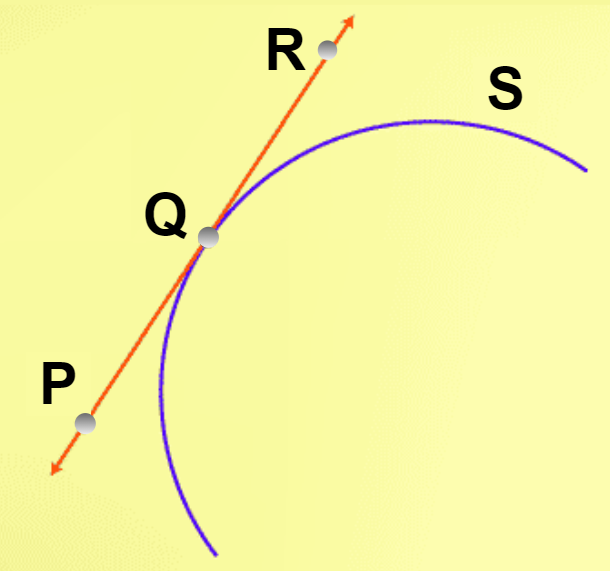## Tangent to a Circle

A line that touches the circle exactly at one point is called the tangent of the circle. We can also define the tangent as the line which tells the direction of the point at a particular point that is performing uniform circular motion around a particular point (centre of the circle).

In the figure below the line is the tangent to the circle which touches the circle at one point on its circumference (P). Thus, any line which touches the curve at a single particular point is called the tangent to that point.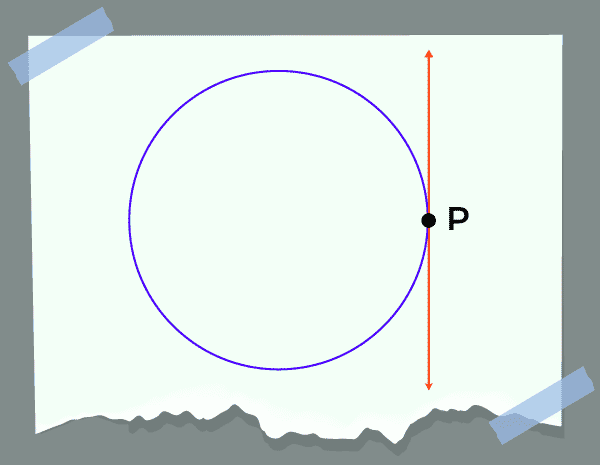Note: A circle can have an infinite number of tangents.

## Point of Tangency

The point where the line touches the curve is called the point of tangency. The tangent to a curve touches the circumference of the curve only at the point of tangency. For the circle point of tangency is the point on the circumference of the circle, from which the tangent line passes. The radius drawn from the point of tangency to the centre of the circle is always perpendicular to the radius of the circle.

• From the above figures, the line is tangent to the circle and the point of tangency is P.

## Equation of Tangent to a Circle

We can easily find the equation of the tangent to a circle. The various equations of tangents are:

• Equation of tangent to a circle with equation x2+ y2 = a2 at point (x1, y1) is

xx1+yy1= a2

• Equation of tangent to a circle with equation x2+ y2+2gx+2fy+c = 0 at point (x1, y1) is

xx1+yy1+g(x+x1)+f(y +y1)+c =0

• Equation of a tangent to a circle with equation x2+ y2 = a2 at point (a cos θ, a sin θ ) is

x cos θ+y sin θ= a

• Equation of a tangent to a circle with equation x2+ y2 = a2  for the line y = mx +c to be tangent is

y = mx ± a √[1+ m2]

## Condition of Tangency

A line is called the tangent to the circle only if touches the circle only at one point else it is simply called the line intersecting the circle. On the basis of the Point of tangency, we divide the condition for tangent into three categories.

• When the point from which the tangent is drawn lies inside the circle then no tangent is formed. The image added below shows the condition when the point at which the tangent is drawn is inside the circle.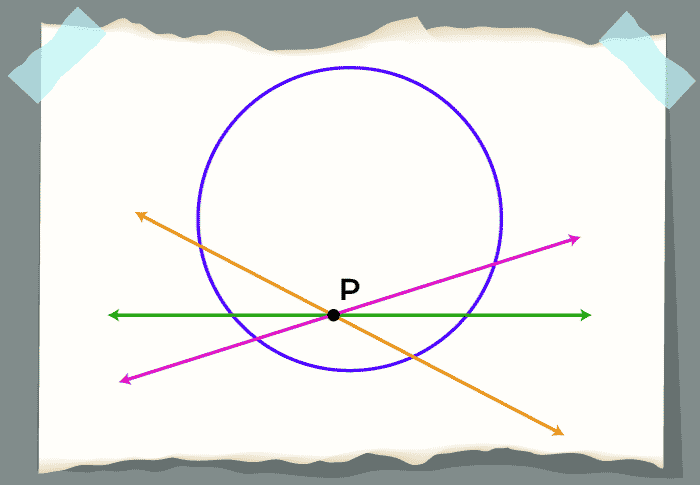• When the point from which the tangent is drawn lies on the circle then only one tangent is possible. The image added below shows the condition when the point at which the tangent is drawn is on the circumference of the circle.• When the point from which the tangent is drawn lies outside the circle then two tangents of equal length are possible. The image added below shows the condition when the point at which the tangent is drawn is outside the circle.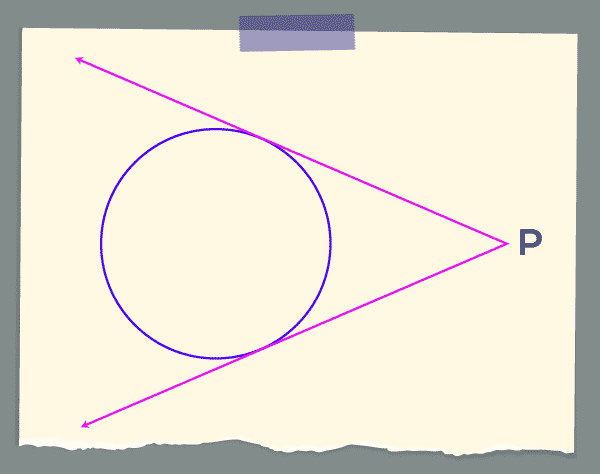## Properties of Tangent

Various properties of the tangent to a circle are

• A tangent only touches the curve at only one point.
• Tangent never enters the circle’s interior and only touches the circumference of the circle once.
• The radius of the circle at the point of tangency is perpendicular to the tangent at that point.
• We can have two tangents to the circle from an exterior point and both tangents are equal in length.

Other than these properties we have various theorems of the tangent that are widely used. Various theorems of the tangents are discussed below in the article.

## Tangent Theorems

We have two important theorems on the tangent of a circle.

• Two Tangents theorem

Statement: The radius at the point of tangency to the circle is perpendicular to the tangent at that point.

Given: Tangent AB to a circle S (with the centre of circle O), and the point of contact is C.

To prove: OC is perpendicular to the tangent AB

Proof: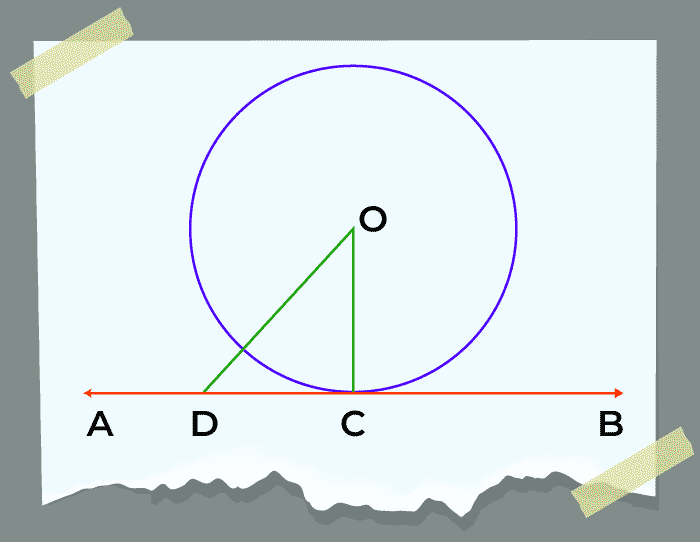Point D lies outside the circle. On joining OD we get OD > OC (radius of a circle). This condition will apply to every point on line AB except point C.

OD > OC proves that OC is the shortest of all the distances of point O to the other points on AB.

Hence proved, OC is perpendicular to AB.

### Two Tangents Theorem

Statement: The statement of the theorem is,

If two tangents are drawn from a point outside the circle then,

• Lengths of these two tangents will be equal, that is, CA = CB.
• Both the tangents will subtend equal angles at the centre, that is, ∠COA = ∠COB.
• Angle between the tangents will be bisected by the line joining the exterior point and the centre, that is, ∠ACO = ∠BCO.

Proof: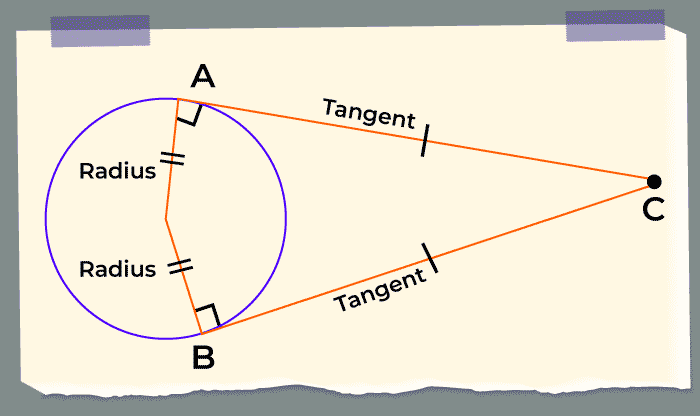All three parts will be proved if we show that ΔCAO is congruent to ΔCBO. Comparing the two triangles, we see that:

OA = OB (radii of the same circle)
OC = OC (common side)
∠OAC = ∠OBC = 90° (Tangent drawn to a circle is perpendicular to the radius at the point of tangency)

Thus, by the RHS criterion, ΔCAO is congruent to ΔCBO, and the truth of all three assertions follows.

## Tangent Formula

If we take any point P outside the circle and draw a tangent at point S on the circumference of the circle. Also, take a secant PQR such that QR is the chord of the circle then,

PS2 = PQ.PR

This is also called the Tangent Secant theorem. We also write this theorem as,

(Tangent)2 = Whole Secant × External Secant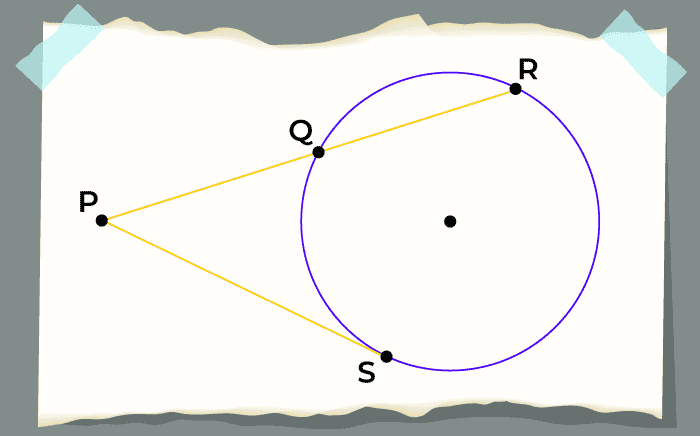Related Resources,

## Solved Examples on Tangents to a Circle

Example 1: In the given circle with centre O and AB is tangent to the circle. The radius of the circle is 5 cm. Find the length of the tangent AB if OP is given to be 13 cm.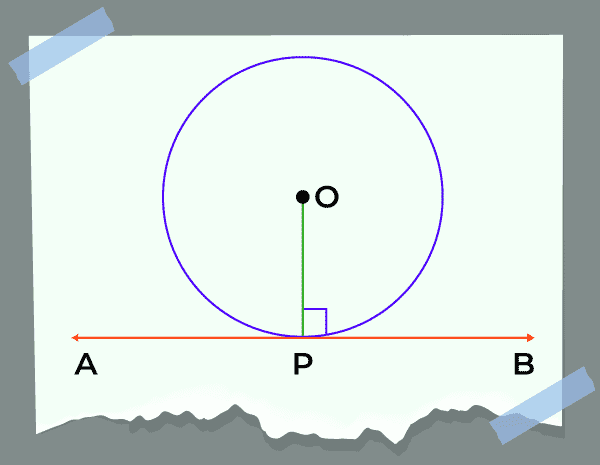Solution:

Given,

AB is tangent to the circle at point O

We know that, tangent AB is perpendicular to the radius OP,

In right triangle ΔOPB using Pythagoras theorem,

OP2 + PB2 = OB2

⇒ PB2 + 52 = 132

⇒ PB2 = 169 – 25 = 144

⇒ PB = √(144) = 12 cm

Length of the tangent AB = 2×PB = 2×12 = 24 cm

Example 2: If PQ and PR are the two tangents to a circle with centre O such that ∠POQ = 110°, then find the value of ∠RPQ.

Solution:

Given,

PQ and PR are tangents to the circle with centre O.

We know that radius is perpendicular to the tangent of the circle.

Thus, OQ is perpendicular to PQ and OR is perpendicular to PR

∠OQP = 90°

⇒ ∠ORP = 90°

Using angle sum property of quadrilaterals.

∠RPQ + ∠QOR + ∠OQP + ∠ORP = 360°

⇒ ∠RPQ = 360° – (110° + 90° + 90°)

⇒ ∠RPQ = 70°

Thus, the value of ∠RPQ is 70°.

their

## FAQs on Tangent to a Circle

### 1. What is the Meaning of Tangent in Circles?

The meaning of the word tangent is ‘to touch’ so it is a line which touches the curve only at point.

### 2. What is Tangent of a Circle?

A line that touches the circle only at a point is called the tangent of the circle. The radius of the circle is always perpendicular to the tangent of the circle.

### 3. What is the Formula for Tangent of a Circle?

The equation of the tangent to the circle x2 + y2 = a2 at point (p, q) is

xp + yq = a2

### 4. What are the Properties of Tangents to a Circle?

Tangent of a circle has various properties some of the important properties of the tangent to a circle are,

• A tangent touches the circle only at one point.
• Radius of the circle is perpendicular to the tangent of the circle.
• Tangents from a external ponint to the circle are always equal

### 5. How do we Know if a line is tangent to a circle?

If the given line touches the circle only at one point on the circumference of the circle then it is called the tangent of the circle.

Whether you're preparing for your first job interview or aiming to upskill in this ever-evolving tech landscape, GeeksforGeeks Courses are your key to success. We provide top-quality content at affordable prices, all geared towards accelerating your growth in a time-bound manner. Join the millions we've already empowered, and we're here to do the same for you. Don't miss out - check it out now!

Previous
Next